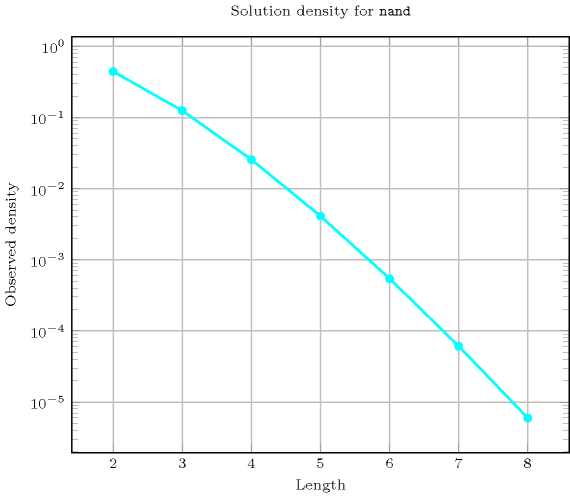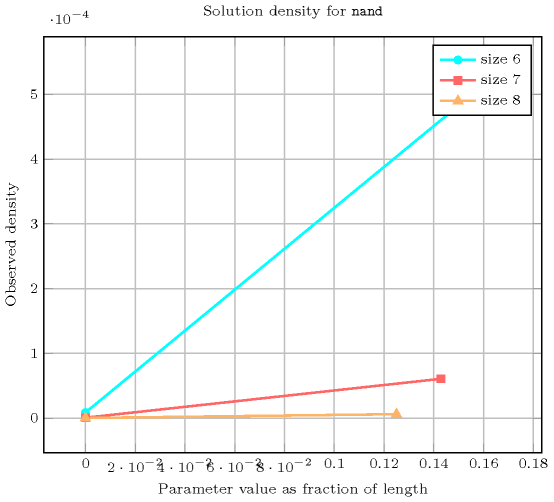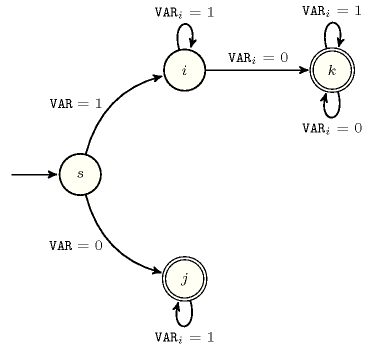## 5.270. nand

Origin

Logic

Constraint

$\mathrm{𝚗𝚊𝚗𝚍}\left(\mathrm{𝚅𝙰𝚁},\mathrm{𝚅𝙰𝚁𝙸𝙰𝙱𝙻𝙴𝚂}\right)$

Synonym

$\mathrm{𝚌𝚕𝚊𝚞𝚜𝚎}$.

Arguments
 $\mathrm{𝚅𝙰𝚁}$ $\mathrm{𝚍𝚟𝚊𝚛}$ $\mathrm{𝚅𝙰𝚁𝙸𝙰𝙱𝙻𝙴𝚂}$ $\mathrm{𝚌𝚘𝚕𝚕𝚎𝚌𝚝𝚒𝚘𝚗}\left(\mathrm{𝚟𝚊𝚛}-\mathrm{𝚍𝚟𝚊𝚛}\right)$
Restrictions
 $\mathrm{𝚅𝙰𝚁}\ge 0$ $\mathrm{𝚅𝙰𝚁}\le 1$ $|\mathrm{𝚅𝙰𝚁𝙸𝙰𝙱𝙻𝙴𝚂}|\ge 2$ $\mathrm{𝚛𝚎𝚚𝚞𝚒𝚛𝚎𝚍}$$\left(\mathrm{𝚅𝙰𝚁𝙸𝙰𝙱𝙻𝙴𝚂},\mathrm{𝚟𝚊𝚛}\right)$ $\mathrm{𝚅𝙰𝚁𝙸𝙰𝙱𝙻𝙴𝚂}.\mathrm{𝚟𝚊𝚛}\ge 0$ $\mathrm{𝚅𝙰𝚁𝙸𝙰𝙱𝙻𝙴𝚂}.\mathrm{𝚟𝚊𝚛}\le 1$
Purpose

Let $\mathrm{𝚅𝙰𝚁𝙸𝙰𝙱𝙻𝙴𝚂}$ be a collection of 0-1 variables ${\mathrm{𝚅𝙰𝚁}}_{1},{\mathrm{𝚅𝙰𝚁}}_{2},\cdots ,{\mathrm{𝚅𝙰𝚁}}_{n}$ $\left(n\ge 2\right)$. Enforce $\mathrm{𝚅𝙰𝚁}=¬\left({\mathrm{𝚅𝙰𝚁}}_{1}\wedge {\mathrm{𝚅𝙰𝚁}}_{2}\wedge \cdots \wedge {\mathrm{𝚅𝙰𝚁}}_{n}\right)$.

Example
 $\left(1,〈0,0〉\right)$ $\left(1,〈0,1〉\right)$ $\left(1,〈1,0〉\right)$ $\left(0,〈1,1〉\right)$ $\left(1,〈1,0,1〉\right)$
Symmetry

Items of $\mathrm{𝚅𝙰𝚁𝙸𝙰𝙱𝙻𝙴𝚂}$ are permutable.

Arg. properties
• Functional dependency: $\mathrm{𝚅𝙰𝚁}$ determined by $\mathrm{𝚅𝙰𝚁𝙸𝙰𝙱𝙻𝙴𝚂}$.

• Contractible wrt. $\mathrm{𝚅𝙰𝚁𝙸𝙰𝙱𝙻𝙴𝚂}$ when $\mathrm{𝚅𝙰𝚁}=0$.

• Extensible wrt. $\mathrm{𝚅𝙰𝚁𝙸𝙰𝙱𝙻𝙴𝚂}$ when $\mathrm{𝚅𝙰𝚁}=1$.

• Aggregate: $\mathrm{𝚅𝙰𝚁}\left(\vee \right)$, $\mathrm{𝚅𝙰𝚁𝙸𝙰𝙱𝙻𝙴𝚂}\left(\mathrm{𝚞𝚗𝚒𝚘𝚗}\right)$.

Counting
 Length ($n$) 2 3 4 5 6 7 8 Solutions 4 8 16 32 64 128 256

Number of solutions for $\mathrm{𝚗𝚊𝚗𝚍}$: domains $0..n$Length ($n$)2345678
Total48163264128256
 Parameter value

01111111
137153163127255

Solution count for $\mathrm{𝚗𝚊𝚗𝚍}$: domains $0..n$Systems

clause in Choco, clause in Gecode, #/\ in SICStus.

Keywords
Cond. implications

$\mathrm{𝚗𝚊𝚗𝚍}\left(\mathrm{𝚅𝙰𝚁},\mathrm{𝚅𝙰𝚁𝙸𝙰𝙱𝙻𝙴𝚂}\right)$

with  $|\mathrm{𝚅𝙰𝚁𝙸𝙰𝙱𝙻𝙴𝚂}|>2$

implies $\mathrm{𝚜𝚘𝚖𝚎}_\mathrm{𝚎𝚚𝚞𝚊𝚕}$$\left(\mathrm{𝚅𝙰𝚁𝙸𝙰𝙱𝙻𝙴𝚂}\right)$.

Automaton

Figure 5.270.1 depicts the automaton associated with the $\mathrm{𝚗𝚊𝚗𝚍}$ constraint. To the first argument $\mathrm{𝚅𝙰𝚁}$ of the $\mathrm{𝚗𝚊𝚗𝚍}$ constraint corresponds the first signature variable. To each variable ${\mathrm{𝚅𝙰𝚁}}_{i}$ of the second argument $\mathrm{𝚅𝙰𝚁𝙸𝙰𝙱𝙻𝙴𝚂}$ of the $\mathrm{𝚗𝚊𝚗𝚍}$ constraint corresponds the next signature variable. There is no signature constraint.

##### Figure 5.270.1. Automaton of the $\mathrm{𝚗𝚊𝚗𝚍}$ constraint##### Figure 5.270.2. Hypergraph of the reformulation corresponding to the automaton of the $\mathrm{𝚗𝚊𝚗𝚍}$ constraint